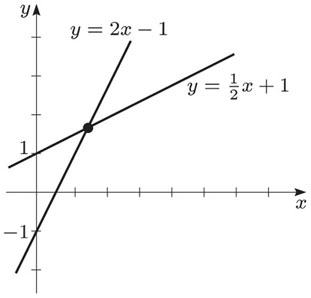# How to Find Point of Intersection of Two Lines

Finding the point of intersection of two lines has many important application such as in Ray-Tracing Simulation.  Two lines always intersect at some point unless they are absolutely parallel, like the rails of a railway track. We start with writing the equations of the two lines in slope-intercept form.

y1=b1+m1*x1

y2=b2+m2*x2Here m1 and m2 are the slopes of the two lines and b1 and b2 are their y-intercepts. At the point of intesection y1=y2, so we have.

b1+m1*x1=b2+m2*x2

But at  the point of intersection x1=x2 as well, so replacing x1 and x2 with x we have.

b1+m1*x=b2+m2*x

or

b1-b2=-x*(m1-m2)

or

x=-(b1-b2)/(m1-m2)

Once the x-component of the point of intersection is found we can easily find the y-component by substituting x in any of the two line equations above.

y=b1+m1*x

In future posts we would like to discuss the cases of intersection of two surfaces and the intersection of two volumes.